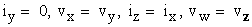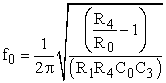﻿ New Very Low Frequency Oscillator Using only a Single CFOA

### New Very Low Frequency Oscillator Using only a Single CFOA

D. K. Srivastava, V. K. Singh, R. SenaniOPEN ACCESSPEER-REVIEWED

## New Very Low Frequency Oscillator Using only a Single CFOA

D. K. Srivastava1, V. K. Singh1,, R. Senani2

1Electronics Engineering Department, Institute of Engineering and Technology, Lucknow, 226021 India

2Division of Electronics and Communication Engineering, NetajiSubhas Institute of Technology, Sector 3, Dwarka, New Delhi, 110078, India

### Abstract

Some time back, Elwakil presented a systematic method of realizing Very Low frequency (VLF) oscillators using current feedback operational amplifiers (CFOA) and demonstrated that the classical Wienbridge oscillator, employing the concept of composite resistor (containing two positive and one negative resistance) can be tailored to generate VLF oscillations. The circuit proposed by Elwakil, however, required two CFOAs along with six resistors and two capacitors. The object of this paper is to report a new VLF oscillator circuit which, in contrast to Elwakil’s circuit, requires onlya single CFOA. The workability of the new circuit has been demonstrated by experimental results using commercially available AD844type CFOAs.

### At a glance: Figures

1
Prev Next

• Srivastava, D. K., V. K. Singh, and R. Senani. "New Very Low Frequency Oscillator Using only a Single CFOA." American Journal of Electrical and Electronic Engineering 3.1 (2015): 1-3.
• Srivastava, D. K. , Singh, V. K. , & Senani, R. (2015). New Very Low Frequency Oscillator Using only a Single CFOA. American Journal of Electrical and Electronic Engineering, 3(1), 1-3.
• Srivastava, D. K., V. K. Singh, and R. Senani. "New Very Low Frequency Oscillator Using only a Single CFOA." American Journal of Electrical and Electronic Engineering 3, no. 1 (2015): 1-3.

 Import into BibTeX Import into EndNote Import into RefMan Import into RefWorks

### 1. Introduction

Very low frequency (VLF) oscillators (capable of generating frequencies < 20Hz) are required in many applications such as bio-medical instrumentation, geo-physical instrumentation and in some measurements in control system applications.

Owing to the well-known advantages of current feedback op-amps (CFOA) (for instance, see [1, 2, 3, 4]) and their wide spread acceptability as alternative building blocks, there have been numerous studies in recent literature on realizing sinusoidal oscillators using CFOAs, for instance, see [5, 6, 7, 8] and references cited therein. In , Elwakil had reported a systematic method of designing VLF oscillators using CFOAs. The circuit of , however, requires two CFOAs along with six resistors and two capacitors (one of which is floating).

The main object of this paper is to introduce a new CFOA-based VLF oscillator, which, by contrast to the circuit of , requires no more than a single CFOA and employs both grounded capacitors, as preferred for integrated circuit implementation.

### 2. Proposed VLF oscillator using a single CFOA

CFOA is a four terminal building block which internally contains a plus type second generation current conveyor  and a voltage follower and is characterized by the following terminal equations(1)

The symbolic notation of the CFOA and its equivalent circuit are shown in Figure 1.

The proposed circuit configuration is shown in Figure 2. Using equation (1) for the CFOA, a straight forward analysis of the circuit gives the condition of oscillation as(2)

whereas the frequency of oscillation is given by(3)

From (2) and (3) it is seen that frequency of oscillation can be adjusted by varying R4 (while, ensuring) whereas the condition of oscillation can also be independently adjusted by C4 and/or R2, out of which using a variable resistance R2 is obviously more preferable. From equation (3), one can see that keeping the difference term in the numerator of FO as small as feasible, generation of VLF oscillations should be possible. It may be mentioned that while designing the circuit, the resistor values should be chosen such that they are much larger than the parasitic x port input impedance Rx (typically around 50Ω) but smaller than the parasitic z port resistance Rp (typically around 3MΩ) of the CFOA. Similarly, the external capacitors have to be chosen such that their values are larger than the parasitic output capacitance Cp (looking into the z terminal) of the CFOA (typically of the order of 4-5pF). This will ensure that the parasitic impedances of the CFOA will not have much effect on the realized frequency of oscillation.

### 3. Experimental Results

The workability of the proposed circuit has been checked by bread-boarded versions of the circuit realized by using AD844 type of CFOAs biased with ±12V DC power supplies, taking R0=R1=1MΩ, C0=C3=100 nF, C4=200 nF with the CO adjusted by variable resistance R2 (1MΩ fixed + 0.0.5M Ω variable). Figure 3 (a) shows the variation of FO with R4, while typical waveform generated from this circuit is shown in Figure 3 (b). The experimental results, thus, confirm the practical workability of the new oscillator circuit.

### 4. Comparison with Previously Known Oscillators

A comparison of the proposed new oscillator with those previously published in literature [5, 6, 7, 8, 10] is now in order. This is shown in Table 1.

Figure 3. Experimental results of the proposed oscillator (a) variation of oscillation frequency with variable resistance R4 (b) a typical waveform generated by the circuit (1.984Hz, 2.5V p-p)

#### Table 1. Comparison with relevant previously published oscillator circuitsDownload asPowerPoint Slide

Veiw figureView current table in a new window

From Table 1, it is seen that the proposed circuit is the only single-CFOA oscillator which possesses all the desirable features while using a reasonable number (only four) of the resistors.

### 5. Concluding Remarks

Anew CFOA-based VLF oscillator circuit configuration has been presented which employs only a single CFOA in contrast to an earlier CFOA-based VLF oscillator proposed by Elwakil  which requires two CFOAs. The workability of the new circuit has been confirmed by experimental results using AD844 type CFOAs and it has been found that the circuit can generate a sinusoidal waveforms of frequency as low as 2 Hz. Lastly, it must be mentioned that although a somewhat similar single CFOA oscillator has been reported earlier in , however, the circuit described here has the advantage of providing independent control of CO also, which is not available in the quoted circuit of .

### Acknowledgements

This work was conducted partly at Analog Signal Processing Research Lab, NSIT, New Delhi and partly at Analog Signal Processing Research Lab, IET, Lucknow. Thanks are due to Prof. D R Bhaskar and Prof. A. K. Singh for their support.

### References

  C.Toumazou and F.J.Lidgey, “Current feedback op-amps: a blessing indisguise?,” IEEECircuits Devices Mag., vol. 10, no.1, pp. 34-37, 1994.In article  F.J.Lidgeyand K.Hayatleh, “Current feedback operational amplifiers and applications, Electron. Commun. Eng, vol. 9, no. 4, pp. 176-182, 1997.In article  A.M. Soliman, “Applications of current feedback amplifier,” Anal. Intregr. Circuits Signal Process. vol. 11, pp. 265-302, 1996.In article  R. Senani, “Realization of a class of analog signal processing/signal generation circuits: novel configurations using current feedback op-amps”, Frequenz, vol. 52, no. 9-10, pp. 196-206, 1998.In article  R. Senani and D. R. Bhaskar, “Single op-amp sinusoidal oscillators suitable for generation of very low frequencies,” IEEE Trans. Instrum. Meas., vol. 40, no. 4, pp. 777-779, 1991.In article  D.R. Bhaskar and R. Senani, “New CFOA-based Single-Element-Controlled Sinusoidal Oscillators,” IEEE Trans. Instrum. Meas. vol. 55, no. 6, pp. 2014-2021, 2006.In article  M. T.Abuelma’atti, “Identification of a class of two CFOA-based sinusoidal RC oscillators,” Analog Integrated Circuits Signal Process., vol. 65, pp. 419-428, 2010.In article  A. S. Elwakil, “Systematic realization of low-frequency oscillators using composite passive-Active resistors”, IEEE Trans. Instrum. Meas., vol. 47, no. 2, pp. 584-586, 1998.In article  A. S. Sedra and K.C. Smith, “A Second generation Current Conveyor and its applications”, IEEE Transaction on Circuit Theory, vol. CT-17, pp. 132-134, February 1970.In article  A. Toker, O. Cicekoglu and H.Kuntman, “On the oscillator implementation using a single current feedback op-amp”, Computers and Electrical Engg., vol. 22, pp. 375-389, 2002.In article